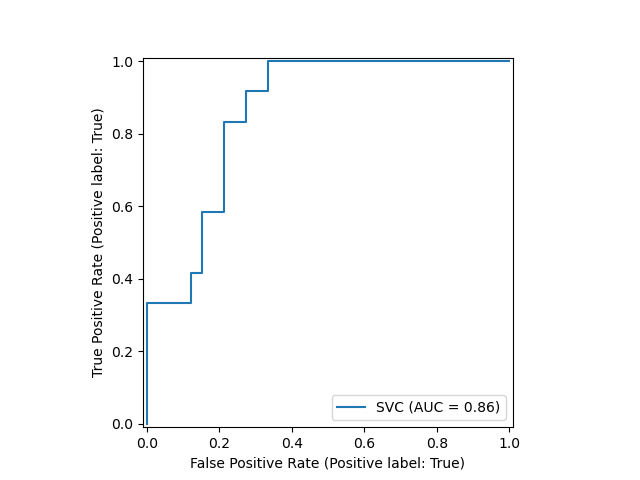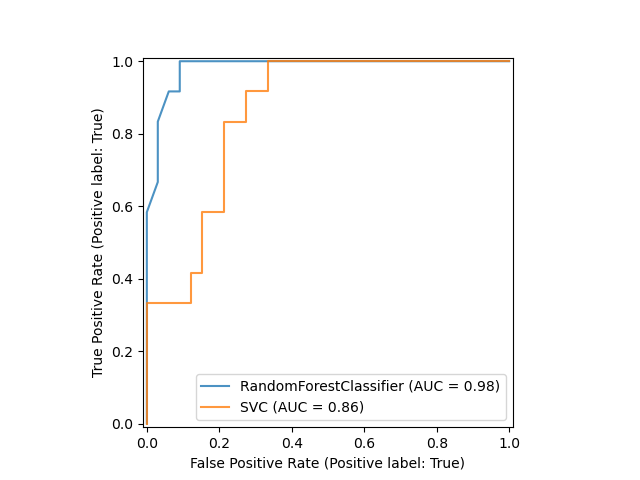# ROC Curve with Visualization API¶

Scikit-learn defines a simple API for creating visualizations for machine learning. The key features of this API is to allow for quick plotting and visual adjustments without recalculation. In this example, we will demonstrate how to use the visualization API by comparing ROC curves.

print(__doc__)


## Load Data and Train a SVC¶

First, we load the wine dataset and convert it to a binary classification problem. Then, we train a support vector classifier on a training dataset.

import matplotlib.pyplot as plt
from sklearn.svm import SVC
from sklearn.ensemble import RandomForestClassifier
from sklearn.metrics import plot_roc_curve
from sklearn.model_selection import train_test_split

y = y == 2

X_train, X_test, y_train, y_test = train_test_split(X, y, random_state=42)
svc = SVC(random_state=42)
svc.fit(X_train, y_train)


Out:

SVC(random_state=42)


## Plotting the ROC Curve¶

Next, we plot the ROC curve with a single call to sklearn.metrics.plot_roc_curve. The returned svc_disp object allows us to continue using the already computed ROC curve for the SVC in future plots.

svc_disp = plot_roc_curve(svc, X_test, y_test)
plt.show()## Training a Random Forest and Plotting the ROC Curve¶

We train a random forest classifier and create a plot comparing it to the SVC ROC curve. Notice how svc_disp uses plot to plot the SVC ROC curve without recomputing the values of the roc curve itself. Furthermore, we pass alpha=0.8 to the plot functions to adjust the alpha values of the curves.

rfc = RandomForestClassifier(n_estimators=10, random_state=42)
rfc.fit(X_train, y_train)
ax = plt.gca()
rfc_disp = plot_roc_curve(rfc, X_test, y_test, ax=ax, alpha=0.8)
svc_disp.plot(ax=ax, alpha=0.8)
plt.show()Total running time of the script: ( 0 minutes 0.170 seconds)

Gallery generated by Sphinx-Gallery